# 5.13 变点理论 · 变点策略初步

## 1.变点理论

CUSUM图作为工业应用检验变点的三大控制图之一，其利用假设检验和极大似然估计的相关统计原理，构建累积和统计量，不断累积观察值与基线水平的差值，将微小偏差累积，放大观察数据出现的波动，从而更加迅速敏感地探测到微小的异常情况，检验出变点位置。其最大的特点是对系统性变化的敏感性，不需要积累太多的样本，因而能较好的控制风险。

## 2.CUSUM原理

CUSUM控制图的设计思想是对信息加以累积，将过程的小偏移累加起来，达到放大的结果，从而提高检验小偏移的灵敏度。CUSUM作为一个统计量，其由来具有严格的数学推理，总的来说，是一个变点假设检验通过极大似然法推导得到的统计量。

`xi`（it）为独立的`N(δ,1)`同分布，其中`t`为未知变点，对于给定的观察序列`xn`，假设`t=v`，如此构成一个假设检验问题: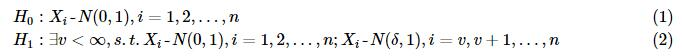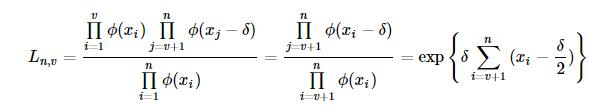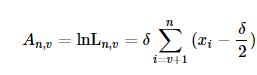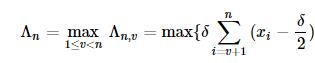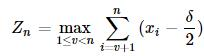`n-1` 个观测值没有均值偏移，即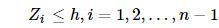`h`为门限。如果在时刻`n`，满足: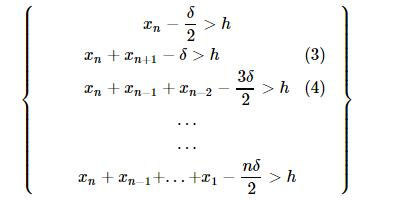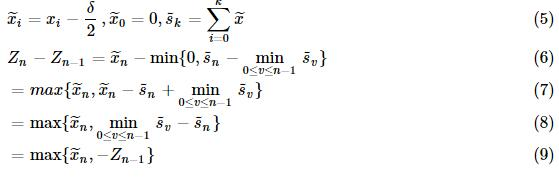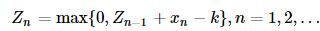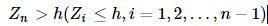## 3.具体应用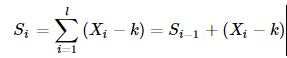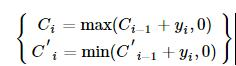``````Ci≥h,C′i≤−h
``````

## 4.参数设定

（1）允偏量k值

2）阈值`h` 在参数检验中，为使得观测数据尽量复合假设，需要将观测到的数据正态标准化，所以阈值h的设置在一定程度上相当于标准正态分布参数的选定，参考布林带的做法，我们将`h`定为2（布林带标准差的倍数一般为2）。

## 5.算法步骤

a) 选取数据段`x0`，计算对数收益率`r`

b) 从数据段`x0`的第一个数据开始，以第一第二个数据为初始数据段`startdata`，进行正态分布拟合，得到均值`mu`和标准差`sigma`，选择数据段外的第一个数据（整体样本的第三个数据），合成实验数据段data，并利用`mu``sigma`值进行归一化，得到标准正态分布序列（近似）`x`

c) 允偏量`k`采取动态变化，为归一化后序列`x`的最后一个数值的一半，计算上下CUSUM统计量，判断`x`序列最后一个数是否超出CUSUM阈值，若超出，对`x`序列最后一个点进行变点标记；若不超出，往后迭代，直至出现变点标记为止；

d) 从`x0`第二个点开始，重现选择数据段，依照a)、b)步骤标记变点，直至标记完所有的起点为止；

e) 若该点存在标记数，则判断该点为变点；

f) 在变点中选择处于股价上升路径的上升变点以及股价处于下降路径中的下降变点，以此确定拐点和拐点方向。 流程图如下：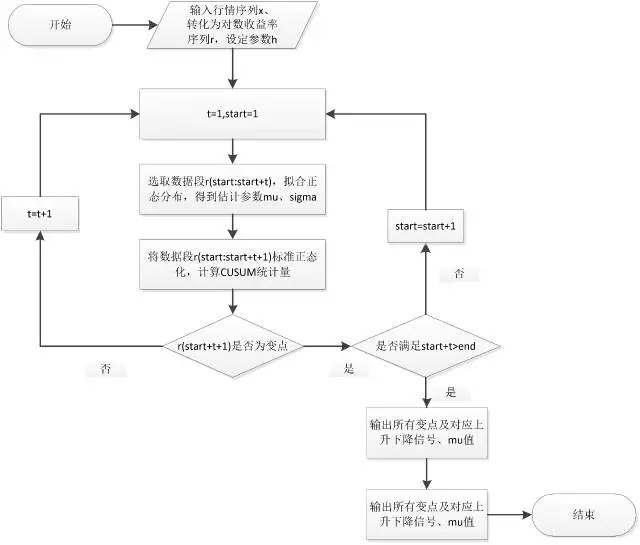## 6.初步程序

``````import numpy as np
import pandas as pd
import scipy.stats as stats
from pandas import Series, DataFrame
price = data1['openPrice'].values                    #取出价格
lnp = np.log(price)                              #取对数
h = 2                                       #设定参数h
R = []                                       #建立一个空列表用于存放对数收益率
i = 1
while i < len(lnp):
r = lnp[i]-lnp[i-1]
i = i+1
R.append(r)                                 #得到对数收益率序列
start = 1
t = 1
while start+t <= len(R)-1:
d = R[(start-1):(start-1+t+1)]
mu,sigma = stats.norm.fit(d)                     #用前面的数据进行正态分布拟合
OR = (R[start-1:start-1+t+1+1]-mu)/sigma             #对该数据段进行标准化
k = OR[-1]/2                                #允偏量设定为归一化后序列的最后一个数值的一半
j = 1
s = 0
while j < t+2:                              #(start-1+t+1+1)-(start-1)=t+2
s = s + OR[j-1]-k                        #计算CUSUM
j = j+1
if s>0:                                     #判断正负
c1 = max(s,0)
if price[start+t] > price[start+t-1] and c1 > h:      #判断是否价格上升并冲破阈值，大于h则买入
print 'c1 = ',c1,start+t                  #start+t即为第(start+t)个变点
start = start+1
elif c1 < h:
t = t+1                             #继续向后迭代直至出现变点为止
elif s<0:
c2 = min(s,0)
if price[start+t] < price[start+t-1] and c2 < -h:     #判断是否价格下降并冲破阈值，小于-h则卖出
print 'c2 = ',c2,start+t
start = start+1
elif c2 > -h:
t = t+1

c2 =  -2.66368724271 3
``````